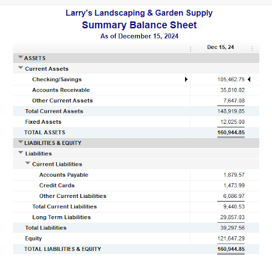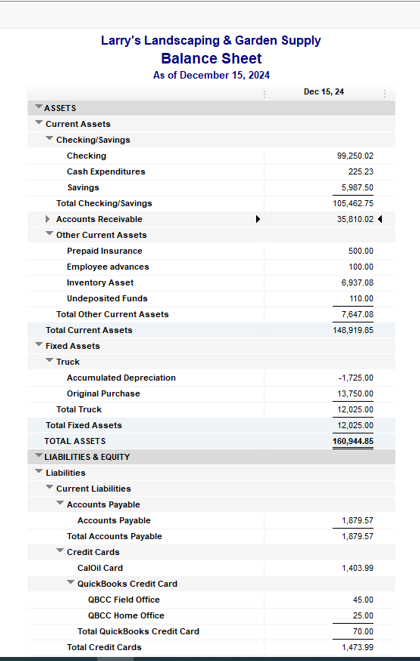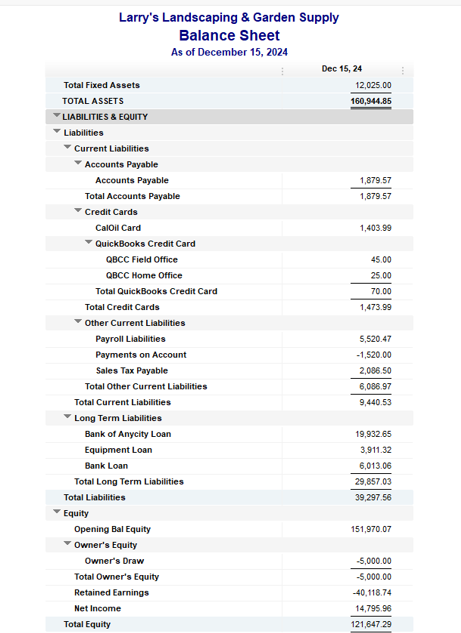# A Beginner's Guide to Quick Ratio

by Mary Girsch-Bock | Published on May 18, 2022

Many or all of the products here are from our partners that pay us a commission. It’s how we make money. But our editorial integrity ensures our experts’ opinions aren’t influenced by compensation. Terms may apply to offers listed on this page.Image source: Getty Images

There are numerous accounting ratios that can be used to determine the financial stability and credit-worthiness of your company.

One of those, the quick ratio, shows the balance between your current assets and your current liabilities, with the best result showing that current company assets outweigh current liabilities. You’ll remember from Accounting 101 that assets are anything you own and liabilities are anything you owe.

While your bookkeeper or staff accountant can certainly calculate a quick ratio, it’s best to let an experienced accountant provide the follow-up analysis on what the quick ratio results mean for your company.

## Overview: What is the quick ratio?

The quick ratio, also referred to as the acid-test ratio, is considered a liquidity ratio. The quick ratio definition is simple: it calculates and measures the ability of your company to pay its current liabilities and debts.

The quick ratio is called such because it only measures liquid assets, or assets that can be quickly converted into cash. You will need to be using double-entry accounting in order to run a quick ratio.

### What is a good quick ratio?

The higher your quick ratio, the better your business will be able to meet any short-term financial obligations. A quick ratio of 1 means that for every \$1 in current liabilities, you have \$1 in current assets.

If the quick ratio for your business is less than 1, it means that your liabilities outweigh your assets, while a quick ratio of 10 means that for every \$1 in liabilities, you have \$10 in liquid assets.

### What’s the difference between the quick ratio vs current ratio?

While both the quick ratio and the current ratio divide assets by liabilities, they differ in the types of assets included in each formula:

• Quick ratio: The quick ratio formula uses current liquid assets, which are assets that can be turned into cash quickly, divided by current liabilities. The quick ratio does not include inventory, prepaid expenses, or supplies in its calculation.
• Current ratio: The current ratio formula is current assets divided by current liabilities, but it includes all current assets, not just liquid assets.

This is an important difference when it comes to determining the ability of your company to pay its short-term liabilities, which is what the quick ratio is designed to do.

## The quick ratio formula

You will use a balance sheet in order to calculate the quick ratio.You can obtain all the information you need to run the quick ratio from your balance sheet. Image source: Author

The quick ratio formula is:

(Cash + Marketable Securities + Accounts Receivable) ÷ Current Liabilities = Quick Ratio

Marketable securities are financial instruments that can be quickly converted to cash, such as government bonds, common stock, and certificates of deposit.

Other assets are excluded from the formula since it calculates your ability to pay debts short-term, so the formula is only concerned with assets that have liquidity.

Current liabilities are short-term debt that are typically due within a year. You should include only current liabilities in your calculation for the same reason listed above; the formula is designed to calculate the ability to pay debts short-term.

## How to calculate the quick ratio

If you’re still confused about how to calculate the quick ratio, we’ll take you through the process step-by-step.

### Step 1: Run a balance sheet

The most important step in the process is running your balance sheet, since you will be pulling all of your numbers from the balance sheet in order to calculate the quick ratio.

Pro Tip: It’s best to run a standard balance sheet, rather than a summary balance sheet, since the standard balance sheet provides both asset and liability details rather than only totals.

### Step 2: Calculate your current assets

Remember, while you want to include current assets in your quick ratio, you only want to include liquid assets.The standard balance sheet provides asset details. Image source: Author

Liquid assets include your cash and accounts receivable, as well as undeposited funds. You would not include inventory or prepaid expenses in the quick ratio calculation, as neither can be converted to cash quickly. To calculate your current assets you will add the following:

 Total Checking/Savings: \$105,462.75 Accounts Receivable: \$ 35,810.02 Undeposited Funds: \$ 110.00 Total liquid assets \$141,382.72

You would not include prepaid insurance, employee advances, and inventory assets since none of those items can be quickly converted to cash.

Pro Tip: Be sure to only include assets that can be quickly converted to cash when calculating the quick ratio.

### Step 3: Calculate your current liabilities

Like your assets, you’ll only want to include your current liabilities when calculating the quick ratio.Only current liabilities should be included in the quick ratio calculation. Image source: Author

Current liabilities include accounts payable, credit card debt, payroll, and sales tax payable, which are all payable within one year. To run the quick ratio, you can use your total current liabilities based on the balance sheet above, which is \$9,440.53. Long term liabilities should be excluded.

Pro Tip: Be sure to exclude long term liabilities such as bank loans when calculating the quick ratio.

### Step 4: Complete the quick ratio calculation

Using the balance sheet totals displayed in Step 2 and Step 3, the numbers you will use to calculate the quick ratio are as follows:

Current assets: \$141,382.77 (Step 2)

Current liabilities: \$9,440.53 (Step 3)

You can now calculate the quick ratio:

\$141,382.77 ÷ \$9,440.53 = 14.98

Pro Tip: Double check your numbers to ensure that only current, liquid assets and current liabilities are included in the quick ratio calculation.

Knowing the quick ratio for your company can help you make needed adjustments such as increasing sales, or developing a more effective accounts receivable collection process.

Knowing the quick ratio can also help when you’re preparing financial projections, no matter what type of accounting your company currently uses.

The quick ratio meaning is simple: does your company have enough current assets to cover its current liabilities. Calculating this ratio can be particularly valuable for those looking to obtain additional financing, as both creditors and investors will want to see how financially stable your company is before lending money.

Finally, if you’re not happy with the results of the quick ratio, you can take steps internally to begin to address the issues that may be causing a low quick ratio, such as the inability to collect on accounts receivable on a timely basis.

Like any ratio, the quick ratio is more beneficial if it’s calculated on a regular basis, so you can determine whether your number is going up down, or remaining the same.

An accurate balance sheet is the key to an accurate quick ratio. If you’re looking for accounting software to help prepare your financial statements, be sure to check out The Ascent’s accounting software reviews.

## FAQs

• The quick ratio lets you know if your company has enough current, liquid assets to pay its short-term debts.

• The quick ratio number is a ratio between assets and liabilities. For instance, a quick ratio of 1 means that for every \$1 of liabilities you have, you have an equal \$1 in assets. A quick ratio of 15 means that for every \$1 of liabilities, you have \$15 in assets.

• If your quick ratio number is too low, you can reexamine company policies, work to increase sales, or institute better collection practices so you can be paid on a more timely basis.